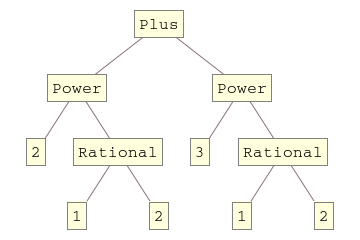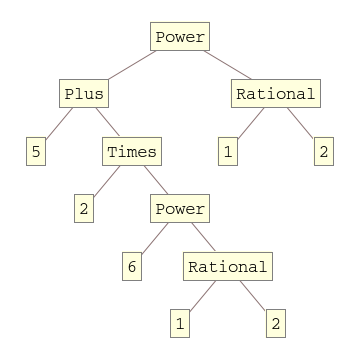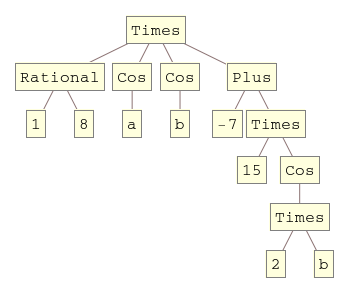## Mathematica – The difference between Equal and SameQ

February 22nd, 2011 | Categories: mathematica, programming | Tags:

A Mathematica user contacted me recently and asked why

(1/16 Cos[a] (Cos[b] + 15 Cos[3 b])) === (1/8 Cos[b] (-7 + 15 Cos[2b])Cos[a])


returned False when he expected True. Let’s take a look at what is going on here.

The SameQ command (===) and the Equal command (==) are subtly different.  Equal (==) tests to see if two expressions are mathematically equivalent whereas SameQ tests to see if they are structurally equivalent.  In general, SameQ is the stricter of the two tests and is often too strict for our needs.

A very simple example

The simplest example might be to compare 2 and 2. (That is 2 with a period at the end)

Mathematically these are the same since they both represent the number two. However, from a Mathematica structural point of view, they are different since one has infinite precision whereas the other has double precision. So, we have

2==2. gives True
2===2. gives False

A more complicated example

Sqrt + Sqrt == Sqrt[5 + 2 Sqrt] which gives True
Sqrt + Sqrt === Sqrt[5 + 2 Sqrt] which gives False


Mathematically the above is always true but the structure of the expressions on the LHS and RHS are different under normal evaluation rules.

So, what do I mean by ‘structurally equivalent?’.  To be honest, I am slightly woolly on this myself but in my head I consider two expressions to be structurally equivalent if their TreeForms are the same

TreeForm[Sqrt + Sqrt ]TreeForm[Sqrt[5 + 2 Sqrt] ]The important thing to remember here is that the argument to TreeForm is evaluated by Mathematica before it gets passed to TreeForm. So, the above images show us that under normal evaluation rules, these two expressions have different structural forms.  In the next example we’ll see how this detail can matter

Exp[I Pi] and all that

Let’s look at

Exp[I Pi] === -1


Would that be True or False based on what we have seen so far?  It is certainly mathematically true but remember that a triple equals requires both sides to be structurally equivalent and at first sight it appears that this isn’t the case here. You might expect Exp[I Pi] to have a very different TreeForm from -1 and so you’d expect the above to evaluate to False. However if you do

TreeForm[Exp[I Pi]]


then you get a single box containing -1. This occurs because Mathematica evaluates Exp[I Pi] to -1 before passing to TreeForm. This evaluation also occurs when you do Exp[I Pi] === -1 so what you are really testing is -1===-1 which is obviously True.

Back to the original example

(1/16 Cos[a] (Cos[b] + 15 Cos[3 b])) === (1/8 Cos[b] (-7 + 15 Cos[2b])Cos[a])


If you use === then it returns False and this is ‘obviously’ the case because if you evaluate the TreeForms of both expressions then you’ll see that they get stored with different structural forms after being evaluated.

TreeForm[(1/16 Cos[a] (Cos[b] + 15 Cos[3 b]))]TreeForm[(1/8 Cos[b] (-7 + 15 Cos[2 b]) Cos[a])]Since we are interested in mathematical equivalence, however, we use ==

(1/16 Cos[a] (Cos[b] + 15 Cos[3 b])) == (1/8 Cos[b] (-7 + 15 Cos[2 b]) Cos[a])


What happens this time, however, is that Mathematica returns the original expression unevaluated. This is because, under the standard set of transformations that Mathematica applies when it evaluates ==, it can’t be sure one way or the other and so it gives up.

To proceed we wrap the whole thing in FullSimplify which essentially says to Mathematica ‘Use everything you’ve got to try and figure this out – no matter how long it takes’  I.e. you allow it to use more transforms.

FullSimplify[(1/16 Cos[a] (Cos[b] + 15 Cos[3 b])) == (1/8 Cos[b] (-7 + 15 Cos[2 b]) Cos[a])]

True


Finally, we have the result that the user expected.

1. Note that there’s special handling for Real objects. Equal ignores last 7 binary digits, SameQ ignores last 1 binary digit. To compare using all digits you can use Order

http://stackoverflow.com/questions/4983885/is-there-a-normal-equalq-function-in-mathematica

2. Note also that you can use it in cases where a variable -might- be set. If you are not sure if a variable is set, you can utilize it to force a true or false.

Remember that Equal not necessarily returns true or false, it can give back an equation. SameQ will always give back true or false:

If[y==2,
x=4
]
If[x===4,
Print[“O hai”]
]

So for the case that y is 2, then x is set, otherwise it isn’t. If x is not set, and you were to use If[x==4, then (most probably) your code will fail, and return an if-statement…

3. Same holds btw for Unequal and UnsameQ:
If you are not sure if someone gives a variable or a value you can use =!= to force a true or false in If statements.